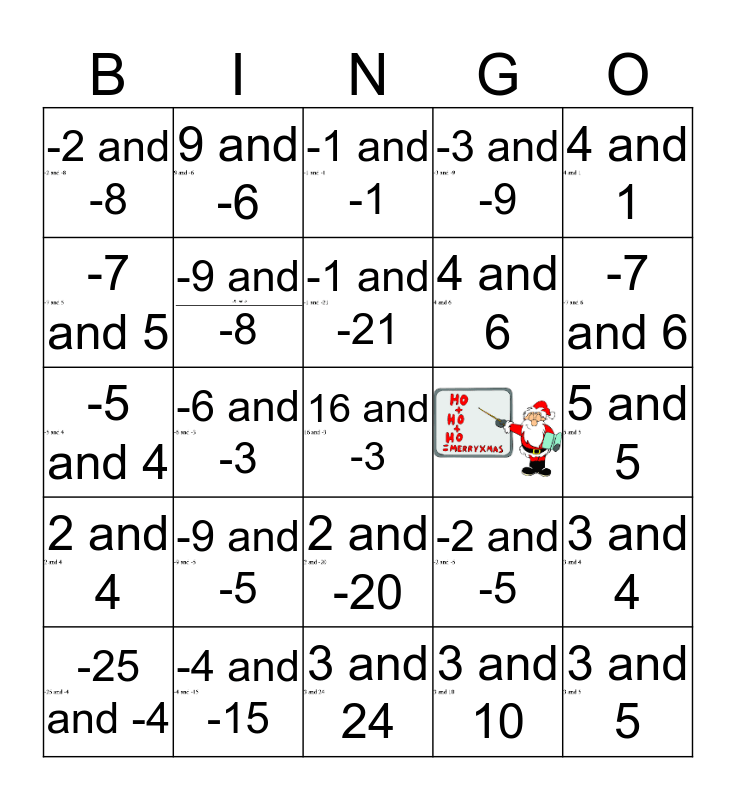# That's Sum Product 2This bingo card has 24 images, a free space and 23 words: -9 and -5, -4 and -15, -1 and -21, -25 and -4, 4 and 1, -2 and -8, -7 and 5, 9 and -6, 2 and -20, 4 and 6, -5 and 4, -2 and -5, 3 and 4, -9 and -8, 3 and 10, 5 and 5, -1 and -1, -7 and 6, -6 and -3, 3 and 5, 2 and 4, -3 and -9 and 3 and 24.

## Play Online